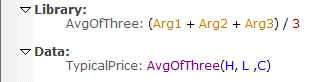Navigation: Realtest Script Language > Script Sections > Library Section

The Library Section and the Data Section look very similar and can, to some degree, be used interchangeably. Their implementation and intended usage are, however, quite different.

The Data Section items are all pre-calculated before any test or scan is run. The calculated values are stored in memory arrays alongside the loaded bar data. Their calculation is extremely fast since it can take advantage of multi-threading, and they can be retrieved from memory very efficiently when accessed since they're stored as fixed-size arrays.

Library Section items, in contrast, are calculated "as needed". So if a formula refers to the same library item twice, it will be calculated twice. Another way to think of Library items is as "formula snippets". It is as if the library item's formula was copied and pasted into the formula that refers to it in place of its name.

While clearly less efficient than the Data Section approach, the advantage here is that a library formula has full access to the context in which it is used. For example, you cannot refer to something like FillPrice or BarsHeld or S.Equity in a Data item. No test has been run yet so there is no context to evaluate these.

In a library item, however, if it is referenced from a strategy formula, then every syntax element available to the strategy is available to the library formula. In other words, library formulas always inherit the context of the formula that refers to them.

See the mr_sample_debug.rts example script for an example of using the Library section.

Library Functions

Library formulas can optionally serve as general-purpose one-line functions.

A Library item can be referenced with up to 9 arguments, as if it were a function, e.g. my_library_item(high, low).

Library items that serve as functions can access the values passed to them by referencing the special build-in variables Arg1 - Arg9.

Here is a contrived example to show how this works:As with any built-in function, the arguments passed to a library function can be any expression (including other library functions).